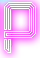# Tjandra 19th birthday present (HARD)

#### Statement

This game/puzzle is about matches, given N matches, your task is to arrange the matches (not necessarily all) such that the number of rectangles (any size) is maximum.

#### Input:

The input begins with the number T of test cases in a single line.

In each of the next T lines there are one integer N.

#### Output:

For each test case, on a single line, print the required answer (maximum number of rectangles).

#### Constraints:

• 1 < T ≤ 100
• 1 < N ≤ 1018

The T numbers N are uniform-randomly chosen in the range.

#### Example input:

```6
3
4
8
12
15
987654321123456789
```

#### Example output:

```0
1
3
9
12
60966316127114768913148159571503206
```

• 0.5 seconds
• 64MB

#### Explanation:

First test case:
No rectangle can be formed with only 3 matches.

Second test case:
Only one rectangle can be formed with 4 matches.

Third test case:
There are max 3 rectangles.
(2 size 1x1, 1 size 2x1) can be formed with number of matches ≤ 8, here is one of the matches formation:Fourth test case:
There are max 9 rectangles.
(4 size 1x1, 2 size 2x1, 2 size 1x2, 1 size 2x2) can be formed with number of matches ≤ 12, here is one of the formation:Fifth test case:
there are max 12 rectangles.
(5 size 1x1, 3 size 2x1, 1 size 3x1, 2 size 1x2, 1 size 2x2) can be formed with number of matches ≤ 15, here is one of the formation:Sixth test case:
You have to figure by yourself how to compute that in the required time.

Problem source: SPOJ